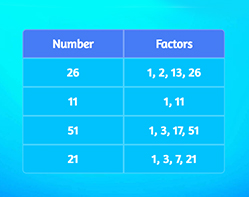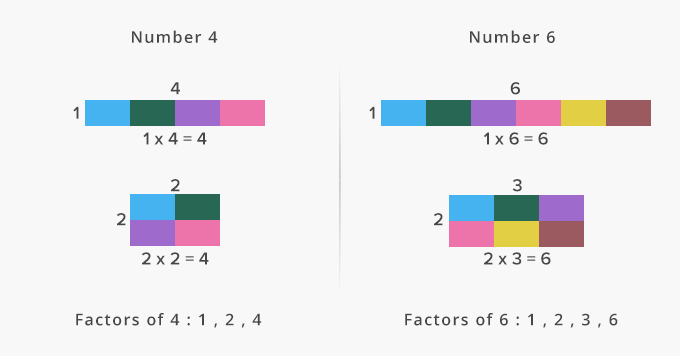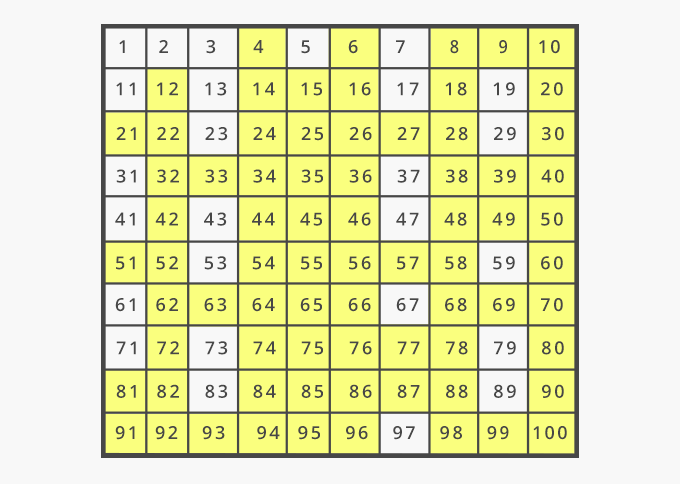# Composite Number - Definition with Examples

The Complete K-5 Math Learning Program Built for Your Child

• 40 Million Kids

Loved by kids and parent worldwide

• 50,000 Schools

Trusted by teachers across schools

• Comprehensive Curriculum

Aligned to Common Core

## Composite Number GamesPrime and Composite Numbers

Identify prime and composite numbers. A prime number has only 2 factors, the number 1 and itself. Remember, that 1 is neither prime nor composite.

Covers Common Core Curriculum 4.OA.4

## What is Composite Number?

In math, composite numbers can be defined as the whole numbers that have more than two factors.
Whole numbers that are not prime are composite numbers, because they are divisible by more than two numbers.

For example, 4, 6, 8, 9 and 10 are the first few composite numbers.Here’s a list of all the composite numbers up to 100, marked in yellow.Fun Facts 1 is neither a prime number nor a compostie number. All even number except 2 are composite numbers. 4 is the smallest composite number.

##Let's sing!

Composite numbers are 4, 6, 8 and 9,
Then, there is also 10 and 12 in line.
They have more than 2 factors on the shelf,
Including the numbers 1 and itself!

OR

Composite numbers are 4, 6, 8 and 9,
Then, there is also 10 and 12 in line.
They have atleast 3 factors on the shelf,
Including the numbers 1 and itself!

##Let's do it!

Ask your children to identify the numbers you say as composite or prime. You can further ask them to find the factors of the composite numbers they identified.

##Related math vocabulary

Won Numerous Awards & Honors